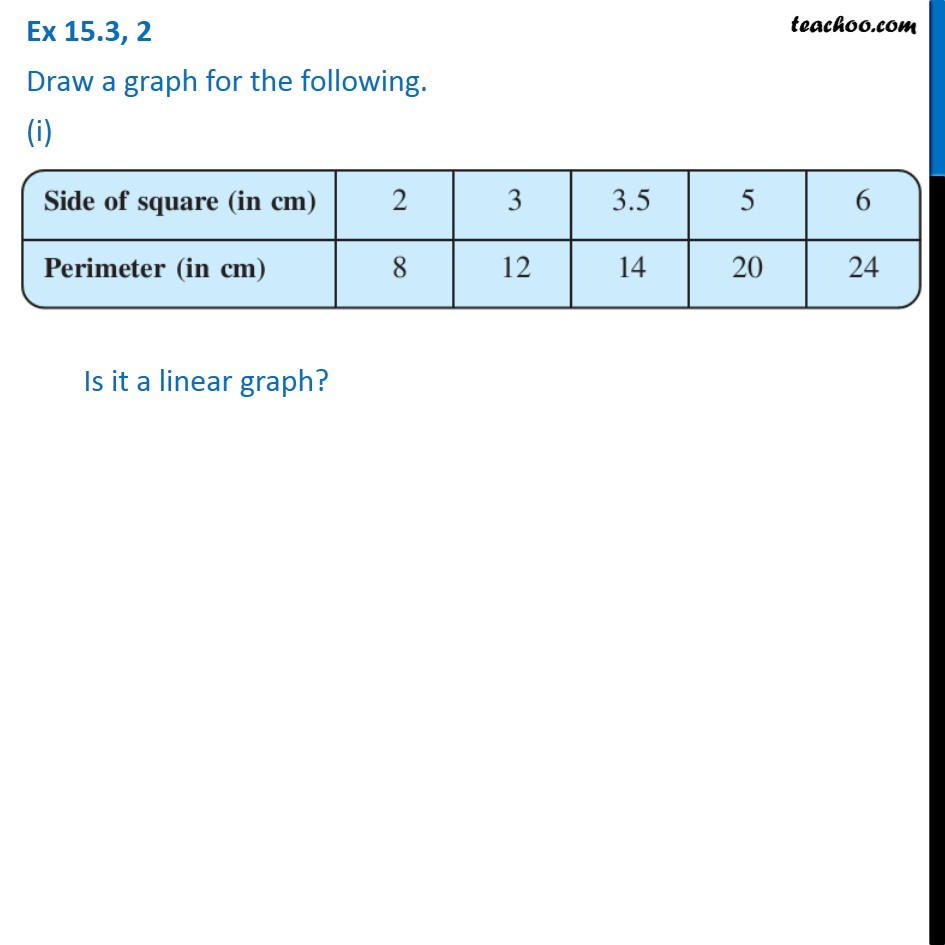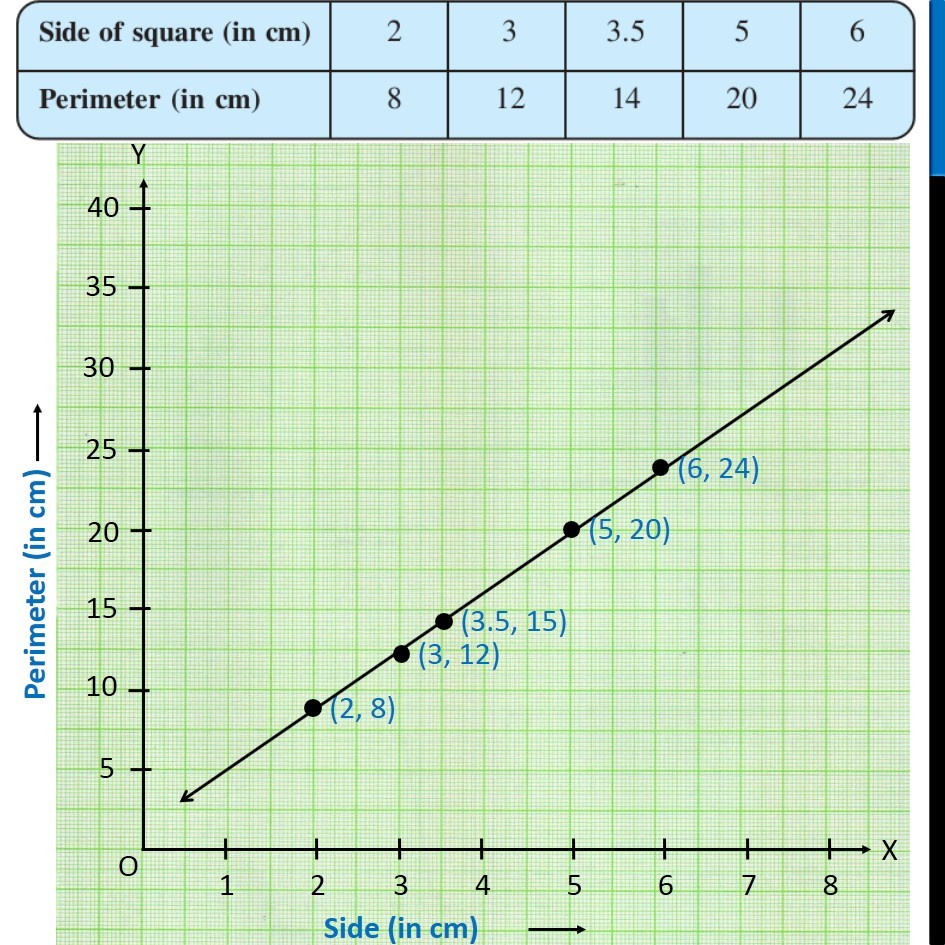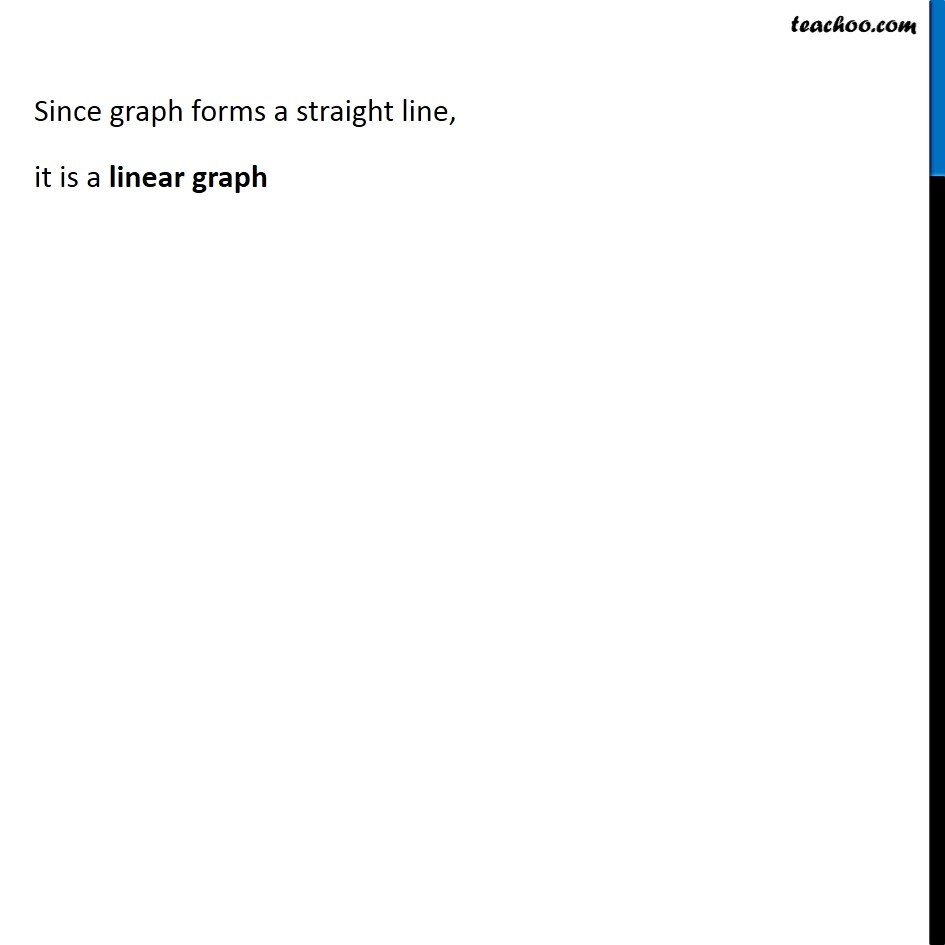Ex 15.3

Chapter 15 Class 8 Introduction to Graphs
Serial order wise### Transcript

Ex 15.3, 2 Draw a graph for the following. (i) Side of square (in cm) | 2 | 3 | 3.5 | 5 | 6 Perimeter (in cm) | 8 | 12 | 14 | 20 | 24 Is it a linear graph? Plotting Perimeter of square in y-axis, from 0 to 40 (with a gap of 5) and Side of square in x-axis, from 0 to 8 (with a gap of 1) Since graph forms a straight line, it is a linear graph